## WitCH 31: Decomposing

We have a short Specialist post coming, and we’ll have more to write on the 2019 VCE exams once they’re online. But, for now, one more Mathematical Methods WitCH, from the 2019 (calculator-free) Exam 1: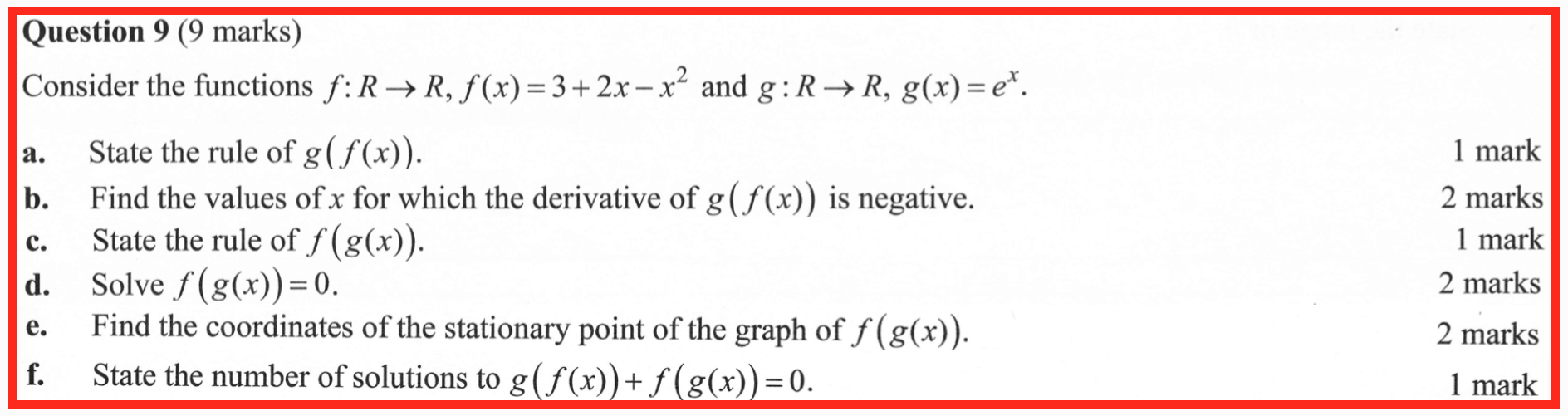This one is double-barrelled. A strange multiple choice question appeared in the 2019 NHT Mathematical Methods Exam 2 (CAS). We had thought to let it pass, but a similar question appeared in last’s weeks Methods exam (no link yet, but the Study Design is here). So, here we go.

First, the NHT question: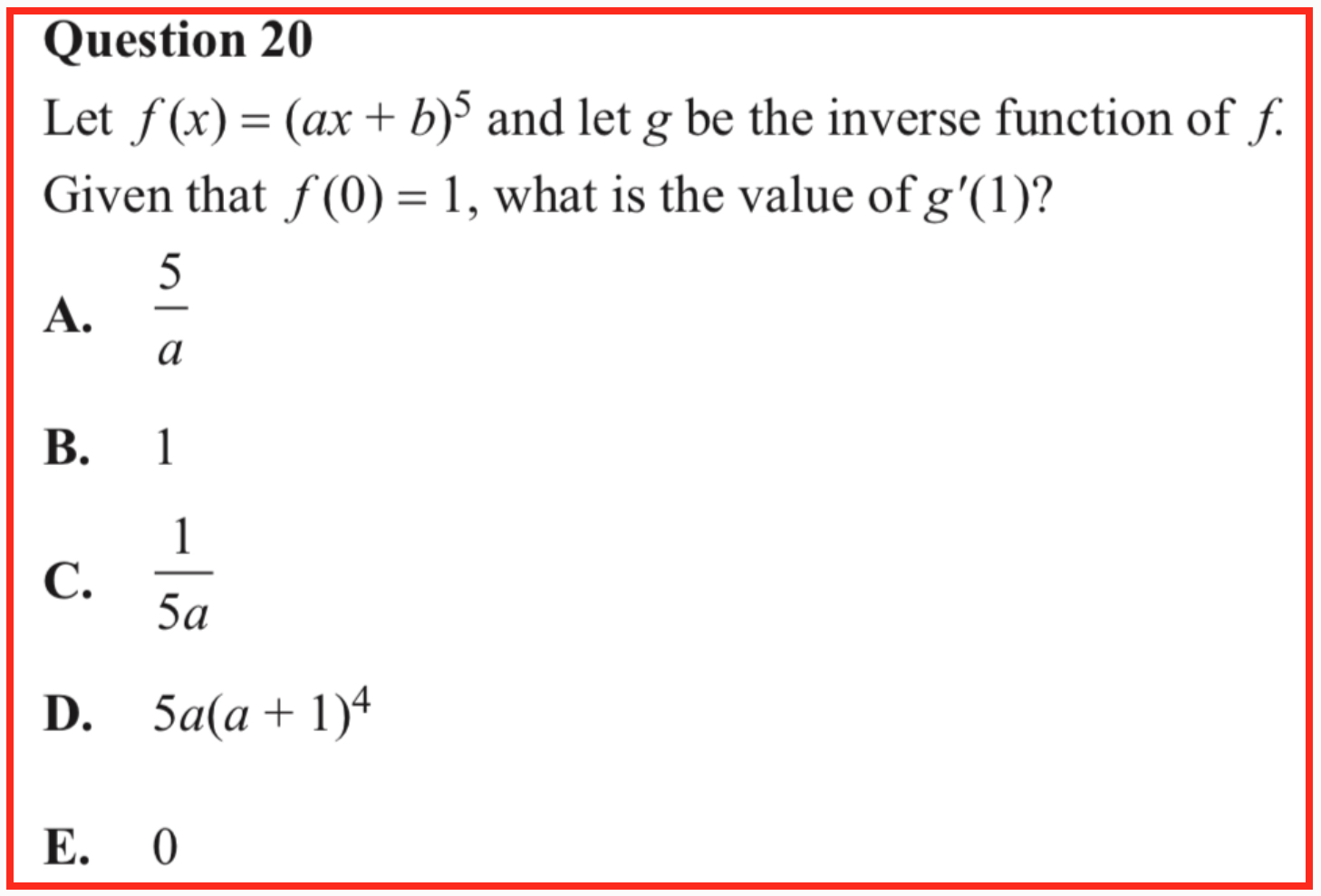The examination report indicates the correct answer, C, and provides a suggested solution:And, here’s last week’s question (with no examination report yet available):## WitCH 27: Uncomposed

Ah, so much crap …

Tons of nonsense to post on, and the Evil Mathologer is breathing down our neck. We’ll have (at least) three posts on last week’s Mathematical Methods exams. This one is by no means the worst to come, but it fits in with our previous WitCH, so let’s quickly get it going. It is from Exam 1. (No link yet, but the Study Design is here.)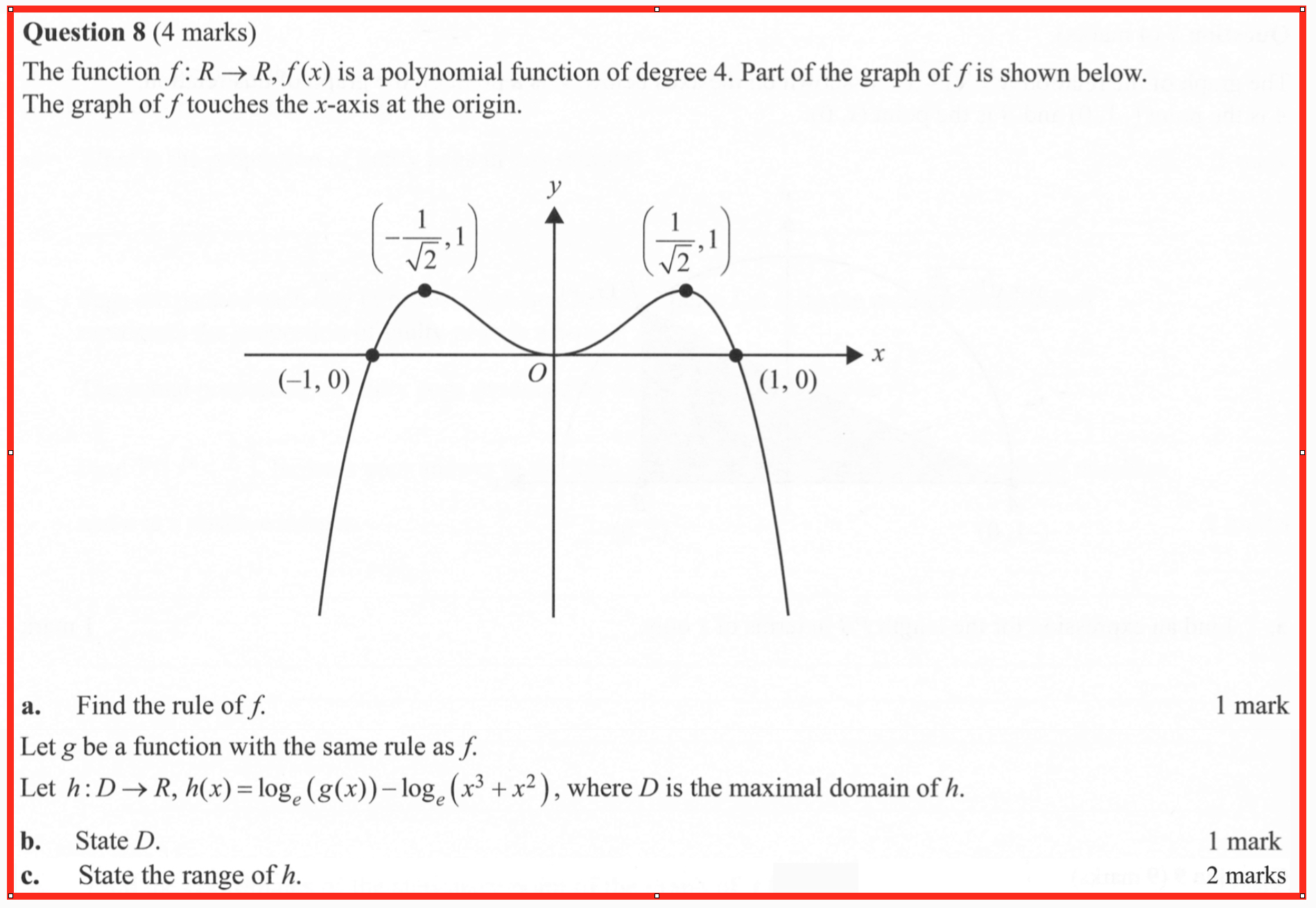## WitCH 26: Imminent Domain

The following WitCH is pretty old, but it came up in a tutorial yesterday, so what the Hell. (It’s also a good warm-up for another WitCH, to appear in the next day or so.) It comes from the 2011 Mathematical Methods Exam 1:For part (a), the Examination Report indicates that f(g)(x) =([x+2][x+8]), leading to c = 2 and d = 8, or vice versa. The Report indicates that three quarters of students scored 2/2, “However, many [students] did not state a value for c and d”.

For Part (b), the Report indicates that 84% of students scored 0/2. After indicating the intended answer, (-∞,-8) U (-2,∞) (-∞,-8] U [-2,∞) or R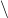(-8,-2), the Report goes on to comment:

“This question was very poorly done. Common incorrect responses included [-3,3] (the domain of  f(x); x ≥ -2 (as the ‘intersection’ of  x ≥ -8 with x ≥ -2); or x ≥ -8 (as the ‘union’ of x ≥ -8 with x ≥ -2). Those who attempted to use the properties of composite functions tended to get confused. Students needed to look for a domain that would make the square root function work.”

The Report does not indicate how students got “confused”, although the composition of functions is briefly discussed in the Study Design (page 72).

## WitCH 10: Malfunction

It’s a long, long time since we’ve had a WitCH. They have been not-so-slowly accumulating, however. And now, since we’re temporarily free of the Evil Mathologer, it is the WitCHing hour.

Due mostly to the hard work of Damo, all of the outstanding WitCHes have been resolved, with the exception of WitCH 8. That one will take time: it’s a jungle of half-maths. Our new WitCHes are not so tricky, although there is perhaps more to be said than indicated at first glance.

The first of our new batch of WitCHes is from the VCE 2018 Specialist Exam 1:The Examiners’ Report gives the answer as. The Report also indicates that the average score on this question was 1.3/5, with 98% of students scoring 3 or lower, and over a third of students scoring 0.

Happy WitCHing.

## Inverted Logic

The 2018 Northern Hemisphere Mathematical Methods exams (1 and 2) are out. We didn’t spot any Magritte-esque lunacy, which was a pleasant surprise. In general, the exam questions were merely trivial, clumsy, contrived, calculator-infested and loathsomely ugly. So, all in all not bad by VCAA standards.

There, was, however, one notable question. The final multiple choice question on Exam 2 reads as follows:

Let f be a one-to-one differentiable function such that f (3) = 7, f (7) = 8, f′(3) = 2 and f′(7) = 3. The function g is differentiable and g(x) = f –1(x) for all x. g′(7) is equal to …

The wording is hilarious, at least it is if you’re not a frazzled Methods student in the midst of an exam, trying to make sense of such nonsense. Indeed, as we’ll see below, the question turned out to be too convoluted even for the examiners.

Of course –1 is a perfectly fine and familiar name for the inverse of f. It takes a special cluelessness to imagine that renaming –1 as g is somehow required or remotely helpful. The obfuscating wording, however, is the least of our concerns.

The exam question is intended to be a straight-forward application of the inverse function theorem. So, in Leibniz form dx/dy = 1/(dy/dx), though the exam question effectively requires the more explicit but less intuitive function form,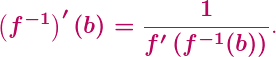IVT is typically stated, and in particular the differentiability of –1 can be concluded, with suitable hypotheses. In this regard, the exam question needlessly hypothesising that the function g is differentiable is somewhat artificial. However it is not so simple in the school context to discuss natural hypotheses for IVT. So, underlying the ridiculous phrasing is a reasonable enough question.

What, then, is the problem? The problem is that IVT is not explicitly in the VCE curriculum. Really? Really.

Even ignoring the obvious issue this raises for the above exam question, the subliminal treatment of IVT in VCE is absurd. One requires plenty of inverse derivatives, even in a first calculus course. Yet, there is never any explicit mention of IVT in either Specialist or Methods, not even a hint that there is a common question with a universal answer.

All that appears to be explicit in VCE, and more in Specialist than Methods, is application of the chain rule, case by isolated case. So, one assumes the differentiability of –1 and and then differentiates –1(f(x)) in Leibniz form. For example, in the most respected Methods text the derivative of y = log(x) is somewhat dodgily obtained using the chain rule from the (very dodgily obtained) derivative of x = ey.

It is all very implicit, very case-by-case, and very Leibniz. Which makes the above exam question effectively impossible.

How many students actually obtained the correct answer? We don’t know since the Examiners’ Report doesn’t actually report anything. Being a multiple choice question, though, students had a 1 in 5 chance of obtaining the correct answer by dumb luck. Or, sticking to the more plausible answers, maybe even a 1 in 3 or 1 in 2 chance. That seems to be how the examiners stumbled upon the correct answer.

The Report’s solution to the exam question reads as follows (as of September 20, 2018):

f(3) = 7, f'(3) = 8, g(x) = f –1(x) , g‘(x) = 1/2 since

f'(x) x f'(y) = 1, g(x) = f'(x) = 1/f'(y).

The awfulness displayed above is a wonder to behold. Even if it were correct, the suggested solution would still bear no resemblance to the Methods curriculum, and it would still be unreadable. And the answer is not close to correct.

To be fair, The Report warns that its sample answers are “not intended to be exemplary or complete”. So perhaps they just forgot the further warning, that their answers are also not intended to be correct or comprehensible.

It is abundantly clear that the VCAA is incapable of putting together a coherent curriculum, let alone one that is even minimally engaging. Apparently it is even too much to expect the examiners to be familiar with their own crappy curriculum, and to be able to examine it, and to report on it, fairly and accurately.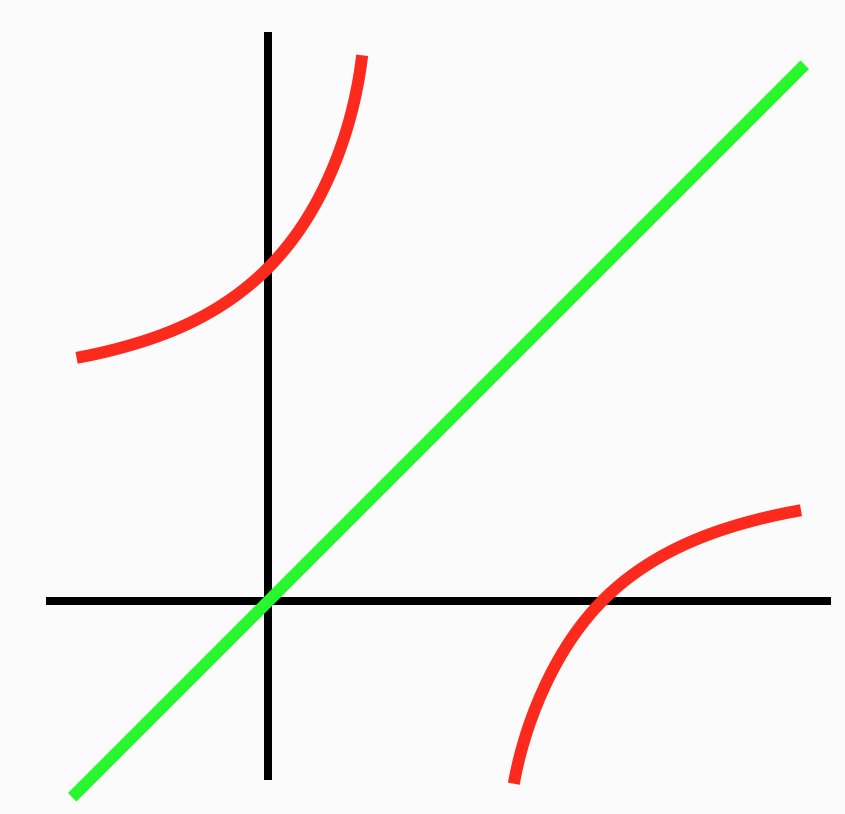Our sixth and final post on the 2017 VCE exam madness is on some recurring nonsense in Mathematical Methods. The post will be relatively brief, since a proper critique of every instance of the nonsense would be painfully long, and since we’ve said it all before.

The mathematical problem concerns, for a given function f, finding the solutions to the equation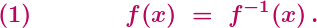This problem appeared, in various contexts, on last month’s Exam 2 in 2017 (Section B, Questions 4(c) and 4(i)), on the Northern Hemisphere Exam 1 in 2017 (Questions 8(b) and 8(c)), on Exam 2 in 2011 (Section 2, Question 3(c)(ii)), and on Exam 2 in 2010 (Section 2, Question 1(a)(iii)).

Unfortunately, the technique presented in the three Examiners’ Reports for solving equation (1) is fundamentally wrong. (The Reports are here, here and here.) In synch with this wrongness, the standard textbook considers four misleading examples, and its treatment of the examples is infused with wrongness (Chapter 1F). It’s a safe bet that the forthcoming Report on the 2017 Methods Exam 2 will be plenty wrong.

What is the promoted technique? It is to ignore the difficult equation above, and to solve instead the presumably simpler equationor perhaps the equationWhich is wrong.

It is simply not valid to assume that either equation (2) or (2)’ is equivalent to (1). Yes, as long as the inverse of f exists then equation (2)’ is equivalent to equation (2): a solution x to (2)’ will also be a solution to (2), and vice versa. And, yes, then any solution to (2) and (2)’ will also be a solution to (1). The converse, however, is in general false: a solution to (1) need not be a solution to (2) or (2)’.

It is easy to come up with functions illustrating this, or think about the graph above, or look here.

OK, the VCAA might argue that the exams (and, except for a couple of up-in-the-attic exercises, the textbook) are always concerned with functions for which solving (2) or (2)’ happens to suffice, so what’s the problem? The problem is that this argument would be idiotic.

Suppose that we taught students that roots of polynomials are always integers, instructed the students to only check for integer solutions, and then carefully arranged for the students to only encounter polynomials with integer solutions. Clearly, that would be mathematical and pedagogical crap. The treatment of equation (1) in Methods exams, and the close to universal treatment in Methods more generally, is identical.

OK, the VCAA might continue to argue that the students have their (stupifying) CAS machines at hand, and that the graphs of the particular functions under consideration make clear that solving (2) or (2)’ suffices. There would then be three responses:

(i) No one tests whether Methods students do anything like a graphical check, or anything whatsoever.

(ii) Hardly any Methods students do do anything. The overwhelming majority of students treat equations (1), (2) and (2)’ as automatically equivalent, and they have been given explicit license by the Examiners’ Reports to do so. Teachers know this and the VCAA knows this, and any claim otherwise is a blatant lie. And, for any reader still in doubt about what Methods students actually do, here’s a thought experiment: imagine the 2018 Methods exam requires students to solve equation (1) for the function f(x) = (x-2)/(x-1), and then imagine the consequences.

(iii) Even if students were implicitly or explicitly arguing from CAS graphics, “Look at the picture” is an absurdly impoverished way to think about or to teach mathematics, or pretty much anything. The power of mathematics is to be able take the intuition and to either demonstrate what appears to be true, or demonstrate that the intuition is misleading. Wise people are wary of the treachery of images; the VCAA, alas, promotes it.

The real irony and idiocy of this situation is that, with natural conditions on the function f, equation (1) is equivalent to equations (2) and (2)’, and that it is well within reach of Methods students to prove this. If, for example, f is a strictly increasing function then it can readily be proved that the three equations are equivalent. Working through and applying such results would make for excellent lessons and excellent exam questions.

Instead, what we have is crap. Every year, year after year, thousands of Methods students are being taught and are being tested on mathematical crap.